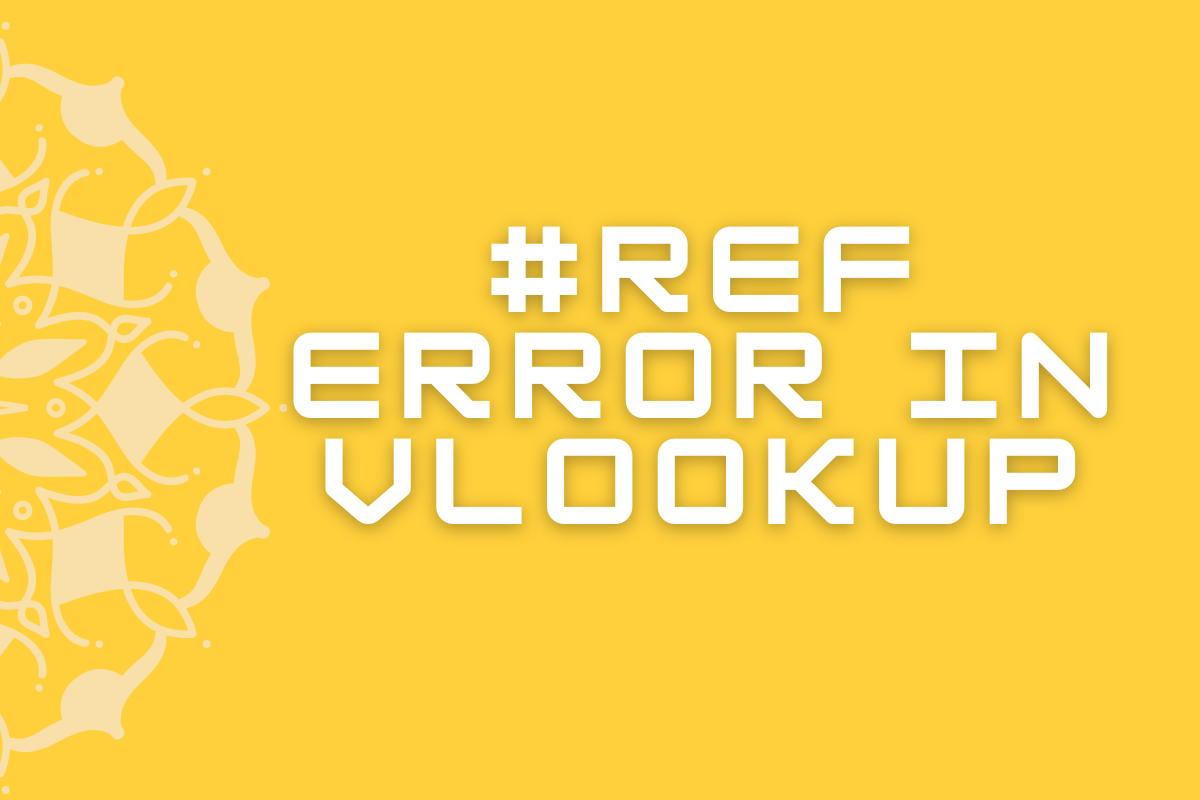# How to Fix #REF Error on VLOOKUP in MS Excel?Formulae are great in-built features in Excel which deliver results of humungous computations in a matter of seconds. But that doesn’t guarantee delivering the correct results every time. There may be times in which errors of some sort shall take up the very place where the results are to be displayed. Errors of such kind are numerous in MS Excel.

The #REF error in VLOOKUP is one such error & we shall look into it elaborately in this article covering both its cause and the steps to tackle it. Following is the dataset that we would be using for the purpose of demonstrating it.

## Why the #REF Error?

Let us first understand why this error happens in order to lay down the strategy to prevent it from happening. As the name indicates, the #REF error has got to do with the cell references given in the formula.

When the data in the cells are no longer available, or the formula has been copied from a different workbook, then the cell references given in the formula will not function as intended.

The need to provide correct cell references is of grave importance while constructing the VLOOKUP formula since its entire functionality depends upon the references that would be given. Let us have a look at its syntax & then get to know about the different entities that require referencing within the formula.

=VLOOKUP( lookup_value, table_array, col_index_num, [range_lookup] )

Where,

• lookup_value – The entity that is to be searched in a tabulated data
• table_array – The tabulated data within which an entity is to be searched
• col_index_num – The count of columns between the position of the entity searched & the position of the desired result.
• range_lookup – To be chosen as zero ‘0’ to return values that actually match the search entity. It’s an optional element which is set to ‘0’ by default.

When each of the above values is not given proper referencing it leads to an error message popping up rather than the result. Let’s see what potential errors can surface in VLOOKUP,

• #N/A Error – When the reference to the lookup_value is incorrect – the search entity is not available in the table array searched. The formula constructed with such reference & the error displayed are given in the subsequent images.
• #REF Error – When the col_index_num is greater than the total number of columns selected for the table array. In the below image it could be seen that only 3 columns have been selected for the table array, but the col_index_num has been given as ‘4’.

Following is the result displayed when one hits ENTER for the above-constructed formula.

## Handling #REF Error:

The #REF error can be sorted out by either adjusting the total count of columns given in the col_index_num of the formula or the total columns selected for the table_array of the formula.

For instance, let us say the intention is to know the Region under which ‘Company J’ falls. So, we correct the VLOOKUP formula’s table range by extending the selected columns to column I which contains the values of Region.

This makes the total number of selected columns to ‘4’ that matches with the col_index_num already given.